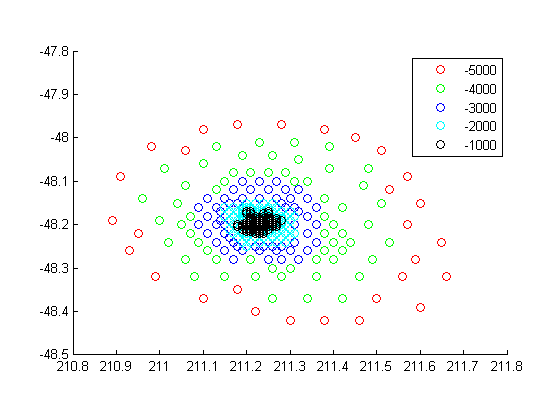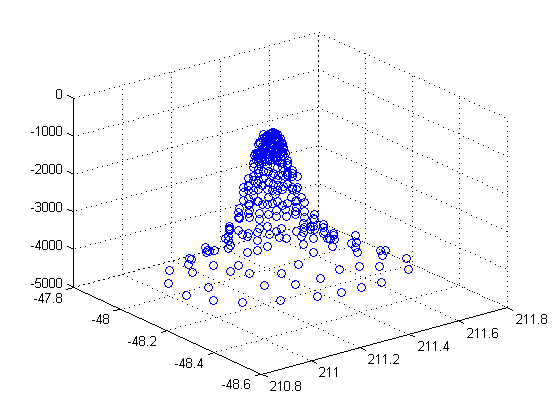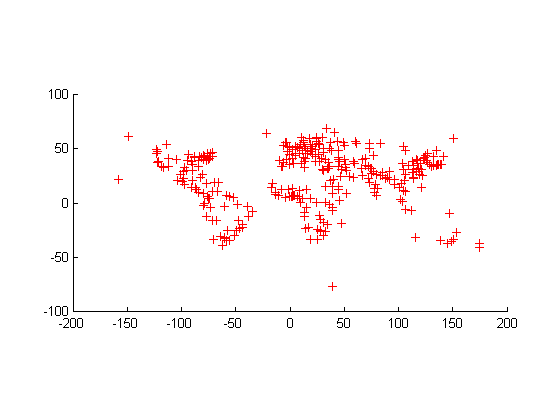# mappoint

Planar point vector

## Description

A mappoint vector is a container object that holds planar point coordinates and attributes. The points are coupled, such that the size of the x- and y-coordinate arrays are always equal and match the size of any dynamically added attribute arrays. Each entry of a coordinate pair and associated attributes, if any, represents a discrete element in the mappoint vector.

To create a planar point shape for use with a geospatial table, create a `mappointshape` object instead.

## Creation

### Syntax

``p = mappoint()``
``p = mappoint(x,y)``
``p = mappoint(x,y,Name,Value)``
``p = mappoint(structArray)``
``p = mappoint(x,y,structArray)``

### Description

example

````p = mappoint()` constructs an empty mappoint vector, `p`, with these default property settings:p = 0x1 mappoint vector with properties: Collection properties: Geometry: 'point' Metadata: [1x1 struct] Feature properties: X: [] Y: []```

example

````p = mappoint(x,y)` constructs a new mappoint vector and assigns the `X` and `Y` properties to the numeric array inputs, `x` and `y`.```

example

````p = mappoint(x,y,Name,Value)` constructs a mappoint vector, then adds dynamic properties to the mappoint vector using `Name`, `Value` argument pairs. You can specify several name-value pair arguments in any order as `Name1,Value1,...,NameN,ValueN`.```

example

````p = mappoint(structArray)` constructs a new `mappoint` vector, assigning the fields of the structure array, `structArray`, as dynamic properties. Field values in `structArray` that are not numeric values, string scalar, string array, character vectors, or cell arrays of numeric values or character vectors are ignored.```

example

````p = mappoint(x,y,structArray)` constructs a new `mappoint` vector, sets the `X` and `Y` properties equal to the numeric arrays `x` and `y`, and sets dynamic properties from the field values of `structArray`.```

## Properties

expand all

Each element in a mappoint vector is considered a feature. For more about the property types in `mappoint`, see Collection Properties and Feature Properties.

Dynamic properties are new features that are added to a mappoint vector and that apply to each individual feature in the mappoint vector. You can attach dynamic Feature properties to a mappoint object during construction with a `Name`,`Value` pair or after construction using dot (`.`) notation. This is similar to adding dynamic fields to a structure. For an example of adding Feature properties dynamically, see Construct a Mappoint Vector for Multiple Features and Examine Autosizing.

Type of geometry, specified as `'point'`. For `mappoint`, `Geometry` is always `'point'`.

Data Types: `char` | `string`

Information for the entire set of mappoint vector elements, specified as a scalar structure. You can add any data type to the structure.

• If `Metadata` is provided as a dynamic property `Name` in the constructor, and the corresponding `Value` is a scalar structure, then `Value` is copied to the `Metadata` property. Otherwise, an error is issued.

• If a `Metadata` field is provided by `structArray`, and both `Metadata` and `structArray` are scalar structures, then the `Metadata` field value is copied to the `Metadata` property value. If `structArray` is a scalar but the `Metadata` field is not a structure, then an error is issued. If `structArray` is not scalar, then the `Metadata` field is ignored.

Data Types: `struct`

Planar x-coordinate, specified as a numeric row or column vector.

Data Types: `double` | `single`

Planar y-coordinates, specified as a numeric row or column vector.

Data Types: `double` | `single`

## Object Functions

 `append` Append features to geographic or planar vector `cat` Concatenate geographic or planar vector `disp` Display geographic or planar vector `fieldnames` Return dynamic property names of geographic or planar vector `isempty` Determine if geographic or planar vector is empty `isfield` Determine if dynamic property exists in geographic or planar vector `isprop` Determine if property exists in geographic or planar vector `length` Return number of elements in geographic or planar vector `properties` Return property names of geographic or planar vector `rmfield` Remove dynamic property from geographic or planar vector `rmprop` Remove property from geographic or planar vector `size` Return size of geographic or planar vector `struct` Convert geographic or planar vector to scalar structure `vertcat` Vertically concatenate geographic or planar vectors

## Examples

collapse all

Dynamically set the `X` and `Y` property values, and dynamically add Vertex property `Z`.

```p = mappoint(); p.X = 1:3; p.Y = 1:3; p.Z = [10 10 10]```
```p = 3x1 mappoint vector with properties: Collection properties: Geometry: 'point' Metadata: [1x1 struct] Feature properties: X: [1 2 3] Y: [1 2 3] Z: [10 10 10]```

Define `x` and `y` coordinates. Use them to create a mappoint.

```x = [40 50 60]; y = [10, 11, 12]; p = mappoint(x, y) ```
```p = 3x1 mappoint vector with properties: Collection properties: Geometry: 'point' Metadata: [1x1 struct] Feature properties: X: [40 50 60] Y: [10 11 12]```
```x = 41:43; y = 1:3; temperature = 61:63; p = mappoint(x, y, 'Temperature', temperature)```
```p = 3x1 mappoint vector with properties: Collection properties: Geometry: 'point' Metadata: [1x1 struct] Feature properties: X: [41 42 43] Y: [1 2 3] Temperature: [61 62 63]```

Create a structure array and then create a mappoint vector, specifying the array as input.

```structArray = shaperead('boston_placenames') p = mappoint(structArray) ```
```structArray = 13x1 struct array with fields: Geometry X Y NAME FEATURE COORD p = 13x1 mappoint vector with properties: Collection properties: Geometry: 'point' Metadata: [1x1 struct] Feature properties: X: [1x13 double] Y: [1x13 double] NAME: {1x13 cell} FEATURE: {1x13 cell} COORD: {1x13 cell```
```[structArray, A] = shaperead('boston_placenames'); x = [structArray.X]; y = [structArray.Y]; p = mappoint(x, y, A)```
```p = 13x1 mappoint vector with properties: Collection properties: Geometry: 'point' Metadata: [1x1 struct] Feature properties: X: [1x13 double] Y: [1x13 double] NAME: {1x13 cell} FEATURE: {1x13 cell} COORD: {1x13 cell}```

This example shows how to add a single feature to an empty mappoint vector after construction.

```x = 1; y = 1; p = mappoint(x, y)```
```p = 1x1 mappoint vector with properties: Collection properties: Geometry: 'point' Metadata: [1x1 struct] Feature properties: X: 1 Y: 1```

Add a dynamic Feature property with a character vector value.

`p.FeatureName = 'My Feature'`
```p = 1x1 mappoint vector with properties: Collection properties: Geometry: 'point' Metadata: [1x1 struct] Feature properties: X: 1 Y: 1 FeatureName: 'My Feature'```

This example show how mappoint vectors autoresize all properties lengths to ensure they are equal in size when a new dynamic property is added or an existing property is appended or shortened.

Create a mappoint vector.

```x = [1 2]; y = [10 10]; p = mappoint(x,y) ```
```p = 2x1 mappoint vector with properties: Collection properties: Geometry: 'point' Metadata: [1x1 struct] Feature properties: X: [1 2] Y: [10 10]```

`p.FeatureName = {'Feature 1','Feature 2'}`
```p = 2x1 mappoint vector with properties: Collection properties: Geometry: 'point' Metadata: [1x1 struct] Feature properties: X: [1 2] Y: [10 10] FeatureName: {'Feature 1' 'Feature 2'}```

Add a numeric dynamic Feature property.

`p.ID = [1 2]`
```p = 2x1 mappoint vector with properties: Collection properties: Geometry: 'point' Metadata: [1x1 struct] Feature properties: X: [1 2] Y: [10 10] FeatureName: {'Feature 1' 'Feature 2'} ID: [1 2]```

Add a third feature. All properties are autosized so that all vector lengths match.

```p(3).X = 3 p(3).Y = 10```
```p = 3x1 mappoint vector with properties: Collection properties: Geometry: 'point' Metadata: [1x1 struct] Feature properties: X: [1 2 3] Y: [10 10 10] FeatureName: {'Feature 1' 'Feature 2' ''} ID: [1 2 0]```

Set the values for the `ID` feature dynamic property with more values than contained in `X` or `Y`. All properties are expanded to match in size.

`p.ID = 1:4`
```p = 4x1 mappoint vector with properties: Collection properties: Geometry: 'point' Metadata: [1x1 struct] Feature properties: X: [1 2 3 0] Y: [10 10 10 0] FeatureName: {'Feature 1' 'Feature 2' '' ''} ID: [1 2 3 4]```

Set the values for the `ID` dynamic Feature property with fewer values than contained in `X` or `Y`. The `ID` property values expand to match the length of `X` and `Y`.

`p.ID = 1:2`
```p = 4x1 mappoint vector with properties: Collection properties: Geometry: 'point' Metadata: [1x1 struct] Feature properties: X: [1 2 3 0] Y: [10 10 10 0] FeatureName: {'Feature 1' 'Feature 2' '' ''} ID: [1 2 0 0]```

Set the values of either coordinate property (`X` or `Y`) with fewer values. All properties shrink in size to match the new length.

```p.X = 1:2 ```
```p = 2x1 mappoint vector with properties: Collection properties: Geometry: 'point' Metadata: [1x1 struct] Feature properties: X: [1 2] Y: [10 10] FeatureName: {'Feature 1' 'Feature 2'} ID: [1 2]```

Remove the `FeatureName` property by setting its value to [ ].

`p.FeatureName = []`
```p = 2x1 mappoint vector with properties: Collection properties: Geometry: 'point' Metadata: [1x1 struct] Feature properties: X: [1 2] Y: [10 10] ID: [1 2]```

Remove all dynamic properties and set the object to empty by setting a coordinate property value to [ ].

```p.X = [] ```
``` Collection properties: Geometry: 'point' Metadata: [1x1 struct] Feature properties: X: [] Y: []```

This example shows how to include multiple dynamic features during object construction.

```point = mappoint([42 44],[10, 11],'Temperature',[63 65], ... 'TemperatureUnits','Fahrenheit') ```
```point = 2x1 mappoint vector with properties: Collection properties: Geometry: 'point' Metadata: [1x1 struct] Feature properties: X: [42 44] Y: [10 11] Temperature: [63 65] TemperatureUnits: 'Fahrenheit'```

This example shows how to construct a mappoint vector using data from a MAT-file containing oceanic depths.

Load data from the `seamount` MAT-file and construct a mappoint vector to hold the coordinates.

```seamount = load('seamount'); p = mappoint(seamount.x, seamount.y, 'Z', seamount.z); ```

Create a level list to use to bin the `z` values and create a list of color values for each level.

```levels = [unique(floor(seamount.z/1000)) * 1000; 0]; colors = {'red','green','blue','cyan','black'};```

Add a `MinLevel` and `MaxLevel` feature property to indicate the lowest and highest binned level. Add a dynamic feature property to indicate the z-coordinate. Add a dynamic Feature property to indicate a binned level value and a color value for a given level. Include metadata information from the MAT-file.

```for k = 1:length(levels) - 1 index = levels(k) <= p.Z & p.Z < levels(k+1); p(index).MinLevel = levels(k); p(index).MaxLevel = levels(k+1) - 1; p(index).Color = colors{k}; end ```

Add metadata information. `Metadata` is a scalar structure containing information for the entire set of properties. You can add any type of data to the structure.

```p.Metadata.Caption = seamount.caption; p.Metadata```
```ans = Caption: [1x229 char] ```

Display the point data as a 2-D plot.

```figure minLevels = unique(p.MinLevel); for k=1:length(minLevels) index = p.MinLevel == minLevels(k); mapshow(p(index).X, p(index).Y, ... 'MarkerEdgeColor', p(find(index,1)).Color, ... 'Marker', 'o', ... 'DisplayType', 'point') end legend(num2str(minLevels')) ```Display the point data as a 3-D scatter plot.

```figure scatter3(p.X, p.Y, p.Z)```This example shows how to create a mappoint vector from a structure array, and how to add features and metadata to the mappoint vector.

```structArray = shaperead('boston_placenames'); p = mappoint(); p.X = [structArray.X]; p.Y = [structArray.Y]; p.Name = {structArray.NAME}```
```p = 13x1 mappoint vector with properties: Collection properties: Geometry: 'point' Metadata: [1x1 struct] Feature properties: X: [1x13 double] Y: [1x13 double] Name: {1x13 cell}```

Construct a mappoint vector from a structure array using the constructor syntax.

```filename = 'boston_placenames.shp'; structArray = shaperead(filename); p = mappoint(structArray)```
```p = 13x1 mappoint vector with properties: Collection properties: Geometry: 'point' Metadata: [1x1 struct] Feature properties: X: [1x13 double] Y: [1x13 double] NAME: {1x13 cell} FEATURE: {1x13 cell} COORD: {1x13 cell}```

Add a `Filename` field to the `Metadata` structure. Display the first five points and the `Metadata` structure.

```p.Metadata.Filename = filename; p(1:5) p.Metadata```
```ans = 5x1 mappoint vector with properties: Collection properties: Geometry: 'point' Metadata: [1x1 struct] Feature properties: X: [2.3403e+05 2.3357e+05 2.3574e+05 2.3627e+05 2.3574e+05] Y: [900038 9.0019e+05 9.0113e+05 9.0097e+05 9.0036e+05] NAME: {1x5 cell} FEATURE: {'PPL-SUBDVSN' ' MARSH' ' HILL' ' PPL' ' PENINSULA'} COORD: {1x5 cell} ans = Filename: 'boston_placenames.shp'```

This example show how to add a feature to the mappoint vector using linear indexing.

Append Paderborn, Germany to the vector of world cities.

```p = mappoint(shaperead('worldcities.shp')); x = 51.715254; y = 8.75213; p = append(p, x, y, 'Name', 'Paderborn'); p(end) ```
```ans = 1x1 mappoint vector with properties: Collection properties: Geometry: 'point' Metadata: [1x1 struct] Feature properties: X: 51.7153 Y: 8.7521 Name: 'Paderborn'```

You can also add a point to the end of the mappoint vector using linear indexing. Add Arlington, Virginia to the end of the vector.

```p(end+1).X = 38.880043; p(end).Y = -77.196676; p(end).Name = 'Arlington'; p(end-1:end)```
```ans = 2x1 mappoint vector with properties: Collection properties: Geometry: 'point' Metadata: [1x1 struct] Feature properties: X: [51.7153 38.8800] Y: [8.7521 -77.1967] Name: {'Paderborn' 'Arlington'} % Plot the points figure mapshow(p.X, p.Y, 'DisplayType', 'point')```This example show how features can be sorted by using the indexing behavior of the mappoint class.

Construct a mappoint vector and sort the dynamic properties.

```p = mappoint(shaperead('tsunamis')); p = p(:, sort(fieldnames(p)))```
```p = 162x1 mappoint vector with properties: Collection properties: Geometry: 'point' Metadata: [1x1 struct] Feature properties: X: [1x162 double] Y: [1x162 double] Cause: {1x162 cell} Cause_Code: [1x162 double] Country: {1x162 cell} Day: [1x162 double] Desc_Deaths: [1x162 double] Eq_Mag: [1x162 double] Hour: [1x162 double] Iida_Mag: [1x162 double] Intensity: [1x162 double] Location: {1x162 cell} Max_Height: [1x162 double] Minute: [1x162 double] Month: [1x162 double] Num_Deaths: [1x162 double] Second: [1x162 double] Val_Code: [1x162 double] Validity: {1x162 cell} Year: [1x162 double]```

Modify the mappoint vector to contain only the dynamic properties, `'Year'`, `'Month'`, `'Day'`, `'Hour'`, `'Minute'`.

`p = p(:, {'Year', 'Month', 'Day', 'Hour', 'Minute'})`
```p = 162x1 mappoint vector with properties: Collection properties: Geometry: 'point' Metadata: [1x1 struct] Feature properties: X: [1x162 double] Y: [1x162 double] Year: [1x162 double] Month: [1x162 double] Day: [1x162 double] Hour: [1x162 double] Minute: [1x162 double]```

Display the first five elements.

`p(1:5)`
```ans = 5x1 mappoint vector with properties: Collection properties: Geometry: 'point' Metadata: [1x1 struct] Feature properties: X: [128.3000 -156 157.9500 143.8500 -155] Y: [-3.8000 19.5000 -9.0200 42.1500 19.1000] Year: [1950 1951 1951 1952 1952] Month: [10 8 12 3 3] Day: [8 21 22 4 17] Hour: [3 10 NaN 1 3] Minute: [23 57 NaN 22 58]```

This example demonstrates that input arguments `x` and `y` can be either row or column vectors.

If you typically store x- and y-coordinate values in an n-by-2 or 2-by-m array, you can assign a mappoint object to these numeric values. If the values are stored in an n-by-2 array, then the `X` property values are assigned to the first column and the `Y` property values are assigned to the second column.

```x = 1:10; y = 21:30; pts = [x' y']; p = mappoint; p(1:length(pts)) = pts```
```p = 10x1 mappoint vector with properties: Collection properties: Geometry: 'point' Metadata: [1x1 struct] Feature properties: X: [1 2 3 4 5 6 7 8 9 10] Y: [21 22 23 24 25 26 27 28 29 30]```

If the values are stored in a 2-by-m array, then the `X` property values are assigned to the first row and the `Y` property values are assigned to the second row.

```pts = [x; y]; p(1:length(pts)) = pts```
```p = 10x1 mappoint vector with properties: Collection properties: Geometry: 'point' Metadata: [1x1 struct] Feature properties: X: [1 2 3 4 5 6 7 8 9 10] Y: [21 22 23 24 25 26 27 28 29 30]```

Observe that in both cases, `X` and `Y` are stored as row vectors.

expand all

## Tips

• If `X`, `Y`, or a dynamic property is set with more values than features in the mappoint vector, then all other properties expand in size using 0 for numeric values and an empty character vector (`''`) for cell values.

• If a dynamic property is set with fewer values than the number of features, then this dynamic property expands to match the size of the other properties, by inserting a 0 if the value is numeric or an empty character vector (`''`), if the value is a cell array.

• If the `X` or `Y` property of the mappoint vector is set with fewer values than contained in the object, then all other properties shrink in size.

• If either `X` or `Y` is set to [ ], then both coordinate properties are set to [ ] and all dynamic properties are removed.

• If a dynamic property is set to [ ], then it is removed from the object.

• The mappoint vector can be indexed like any MATLAB® vector. You can access any element of the vector to obtain a specific feature. The following examples demonstrate this behavior:

## Version History

Introduced in R2012a